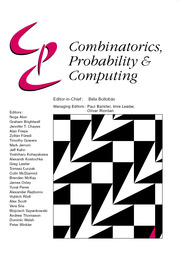Home
Hostname: page-component-79b67bcb76-6j5sx Total loading time: 0.203 Render date: 2021-05-13T05:21:40.150Z Has data issue: true Feature Flags: { "shouldUseShareProductTool": true, "shouldUseHypothesis": true, "isUnsiloEnabled": true, "metricsAbstractViews": false, "figures": false, "newCiteModal": false, "newCitedByModal": true, "newEcommerce": true }Combinatorics, Probability and Computing

# Additive and Multiplicative Structure in Matrix Spaces

Published online by Cambridge University Press:  01 March 2007

Corresponding

## Abstract

Let A be a set of N matrices. Let g(A) ≔ |A + A| + |A · A|, where A + A = {a1 + a2aiA} and A · A = {a1a2aiA} are the sum set and product set. We prove that if the determinant of the difference of any two distinct matrices in A is nonzero, then g(A) cannot be bounded below by cN for any constant c. We also prove that if A is a set of d × d symmetric matrices, then there exists ϵ = ϵ(d)>0 such that g(A)>N1+ϵ. For the first result, we use the bound on the number of factorizations in a generalized progression. For the symmetric case, we use a technical proposition which provides an affine space V containing a large subset E of A, with the property that if an algebraic property holds for a large subset of E, then it holds for V. Then we show that the system a2 : aV is commutative, allowing us to decomposeas eigenspaces simultaneously, so we can finish the proof with induction and a variant of the Erdős–Szemerédi argument.

Type
Paper
Information
Combinatorics, Probability and Computing , March 2007 , pp. 219 - 238

## Access options

Get access to the full version of this content by using one of the access options below.

## References

Bilu, Y. (1999) Structure of sets with small sumset. Structure Theory of Set Addition: Astérisque 258 pp. 77108.Google Scholar
Bourgain, J. and Chang, M.-C. (2004) On the size of k-fold sum and product sets of integers. J. Amer. Math. Soc. 17 pp. 473497.CrossRefGoogle Scholar
Bourgain, J., Katz, N. and Tao, T. (2004) A sum-product estimate in finite fields, and applications. GAFA 14 pp. 2757.Google Scholar
Chang, M.-C. (2002) Factorization in generalized arithmetic progressions and applications to the Erdős–Szemerédi sum-product problems. GAFA 113 pp. 399419.Google Scholar
Chang, M.-C. (2003) Erdős–Szemerédi problem on sum set and product set. Annals of Math. 157 939957.CrossRefGoogle Scholar
Chang, M.-C. (2004) On sums and products of distinct numbers. J. Combin. Theory Ser. A 105 349354.CrossRefGoogle Scholar
Chang, M.-C. (2004) A sum-product theorem in semi-simple commutative Banach algebras. J. Funct. Anal. 212 399430.CrossRefGoogle Scholar
Chang, M.-C. (2005) A sum-product estimate in algebraic division algebras over R. Israel J. Math. 150 pp. 369380.CrossRefGoogle Scholar
Elekes, G. (1997) On the number of sums and products. Acta Arithmetica 81 365367.CrossRefGoogle Scholar
Elekes, G. and Ruzsa, I. Z. (2003) Product sets are very large if sumsets are very small. Studia Sci. Math. Hungar. 40.Google Scholar
Erdős, P. and Szemerédi, E. (1983) On sums and products of integers. In Studies in Pure Mathematics, Birkhäuser, Basel, pp. 213218.CrossRefGoogle Scholar
Ford, K. (1998) Sums and products from a finite set of real numbers. Ramanujan J. 2 5966.CrossRefGoogle Scholar
Nathanson,, M. B. (1993) The simplest inverse problems in additive number theory. In Number Theory with an Emphasis on the Markoff Spectrum (Pollington, A. and Moran, W., eds), Marcel Dekker, pp. 191206.Google Scholar
Nathanson, M. B. (1996) Additive Number Theory: Inverse Problems and the Geometry of Sumsets, Springer.CrossRefGoogle Scholar
Nathanson, M. B. (1997) On sums and products of integers. Proc. Amer. Math. Soc. 125 916.CrossRefGoogle Scholar
Nathanson, M. and Tenenbaum, G. (1999) Inverse theorems and the number of sums and products. Structure Theory of Set Addition: Astérisque 258 195204.Google Scholar
Ruzsa, I. Z. (1994) Generalized arithmetic progressions and sumsets. Acta Math. Hungar. 65 379388.CrossRefGoogle Scholar
Solymosi, J. (2005) On the number of sums and products. Bulletin London Math. Soc. (4) 37 pp. 491494.CrossRefGoogle Scholar

# Send article to Kindle

Note you can select to send to either the @free.kindle.com or @kindle.com variations. ‘@free.kindle.com’ emails are free but can only be sent to your device when it is connected to wi-fi. ‘@kindle.com’ emails can be delivered even when you are not connected to wi-fi, but note that service fees apply.

Find out more about the Kindle Personal Document Service.

Additive and Multiplicative Structure in Matrix Spaces
Available formats
×

# Send article to Dropbox

To send this article to your Dropbox account, please select one or more formats and confirm that you agree to abide by our usage policies. If this is the first time you use this feature, you will be asked to authorise Cambridge Core to connect with your <service> account. Find out more about sending content to Dropbox.

Additive and Multiplicative Structure in Matrix Spaces
Available formats
×

# Send article to Google Drive

To send this article to your Google Drive account, please select one or more formats and confirm that you agree to abide by our usage policies. If this is the first time you use this feature, you will be asked to authorise Cambridge Core to connect with your <service> account. Find out more about sending content to Google Drive.

Additive and Multiplicative Structure in Matrix Spaces
Available formats
×
×